## RS Aggarwal Class 6 Solutions Chapter 10 Ratio, Proportion and Unitary Method Ex 10C

These Solutions are part of RS Aggarwal Solutions Class 6. Here we have given RS Aggarwal Solutions Class 6 Chapter 10 Ratio, Proportion and Unitary Method Ex 10C.

Other Exercises

Question 1.
Solution:
Cost of 14 m of cloth = Rs. 1890
Cost of 1 m = Rs. $$\\ \frac { 1890 }{ 14 }$$
and cost of 6 m = Rs. $$\\ \frac { 1890\times 6 }{ 14 }$$
= Rs. 135 x 6
= Rs. 810

Question 2.
Solution:
Cost of 1 dozen or 12 soaps = Rs. 285.60
Cost of 1 soap = Rs. $$\\ \frac { 285.60 }{ 12 }$$
Cost of 15 soaps = Rs. $$\\ \frac { 285.60\times 15 }{ 12 }$$
= Rs. 357.00

Question 3.
Solution:
Cost of 9 kg of rice = Rs. 327.60
Cost of 1 kg = Rs. $$\\ \frac { 327.60 }{ 9 }$$
and cost of 50 kg = Rs. $$\\ \frac { 327.60\times 50 }{ 9 }$$
= Rs. 36.40 x 50
= Rs. 1820

Question 4.
Solution:
Weight of 22.5 metres of the iron rod: = 85.5 kg
Weight of 1 metre of the iron rod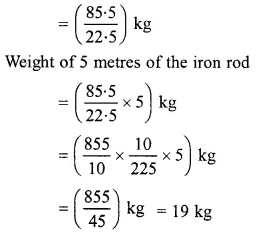Question 5.
Solution:
Quantity of oil in 15 tins = 234 kg
Quantity of oil in 1 tin = $$\\ \frac { 234 }{ 15 }$$ kg
Quantity of oil in 10 tinsQuestion 6.
Solution:
Distance covered by the car in 12 litres of diesel = 222 kms
Distance covered by the car in 1 litre of diesel = $$\\ \frac { 222 }{ 12 }$$ km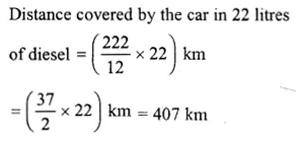Question 7.
Solution:
Charges of 25 tonnes of weight = Rs. 540
charges of 1 ton = Rs.$$\\ \frac { 540 }{ 25 }$$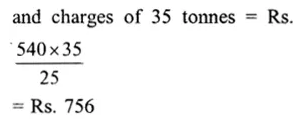Question 8.
Solution:
Weight of copper in 4.5 g of alloy = 3.5g
Weight of copper in 1 g of alloy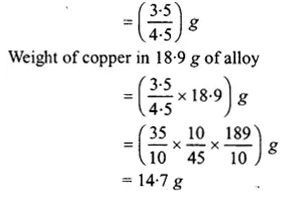Question 9.
Solution:
In Rs. 87.50, the inland letter are purchased = 35
In Re. 1, letters can be purchased
= $$\\ \frac { 35 }{ 87.50 }$$
and in Rs. 315, letters can be purchasedQuestion 10.
Solution:
4 dozen = 4 x 12 = 48 bananas
In Rs. 104, banana are purchased = 48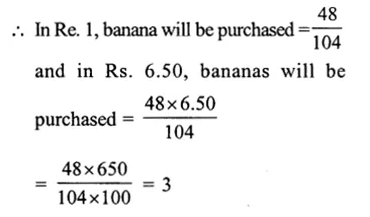Question 11.
Solution:
In Rs. 22770, chairs are purchased =18
In Re. 1, chairs will be purchased
= $$\\ \frac { 18 }{ 22770 }$$
and in Rs. 10120, chairs will be
purchased = $$\\ \frac { 18\times 10120 }{ 22770 }$$
= 8

Question 12.
Solution:
(i) A car travels 195 km distance in = 3 hours
It will travel 1 km distance in = $$\\ \frac { 3 }{ 195 }$$ hr.
and it will travel 520 km distance in
= $$\\ \frac { 3\times 520 }{ 195 }$$
= 8 hr
(ii) A car travels in 3hr = 195 km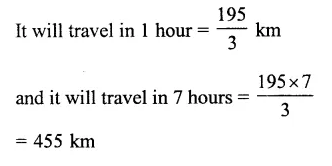Question 13.
Solution:
(i) A laborer earn in 12 days = Rs. 1980
He will earn in 1 day = Rs. $$\\ \frac { 1980 }{ 12 }$$
and he will earn in 7 days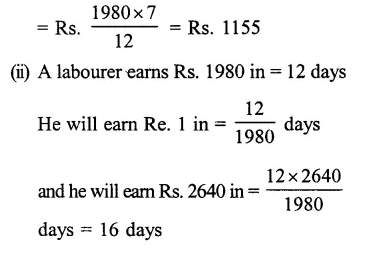Question 14.
Solution:
(i) Weight of 65 books = 13 kg
Then weight of 1 book = $$\\ \frac { 13 }{ 65 }$$ kg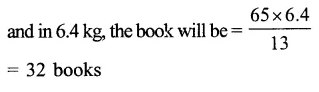Question 15.
Solution:
Number of boxes needed for 6000 pens = 48
Number of boxes needed for 1 pen 48 = $$\\ \frac { 48 }{ 6000 }$$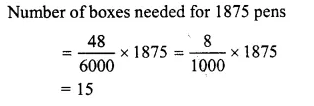Question 16.
Solution:
Clearly, less workers will build the wall in more days.
And, more workers will build the wall in less days.
24 workers can build the wall in 15 days
1 worker can build the wall in (15 x 24) days
(less worker, more days)
9 workers will build the wall inQuestion 17.
Solution:
Men needed to finish a piece of work in 26 days = 40
Men needed to finish a piece of work in 1 day = 40 x 26 (less days, more men)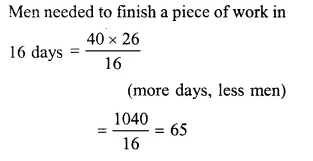Question 18.
Solution:
Clearly, less men will take more days to consume the food.
And, more men will take less days to consume the food.
550 men have provisions for 28 days
1 men has provisions for (28 x 550) days [less men, more days]
700 men will have provisions for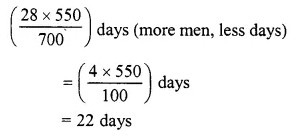Question 19.
Solution:
Clearly, less persons will consume the rice in more days.
And more persons will consume the rice in less days.
60 persons consume the bag of rice in 3 days.
1 person will consume the bag of rice in
(3 x 60) days (less persons, more days)
18 persons will consume the bag of rice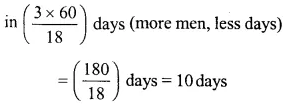Hope given RS Aggarwal Solutions Class 6 Chapter 10 Ratio, Proportion and Unitary Method Ex 10C are helpful to complete your math homework.

If you have any doubts, please comment below. Learn Insta try to provide online math tutoring for you.